# Compendium of Chinese-Rings-Like Puzzles

## 3 Definitions, Examples and More

This compendium contains a special collection of puzzles which are somehow related to the famous Chinese Rings puzzle. For a definition which puzzles are included in this compendium and for a clear understanding why they are included, we will provide a structural definition of the class of puzzles in this compendium, the CR recursive puzzles. We will provide a definition, with some examples, and with some interesting properties of these puzzles.

### 3.1 Definition of CR Recursive Puzzle

 A CR recursive puzzle is a puzzle that contains m special similar pieces (with m ≥ 1) and the puzzle can be generalized to other values of m ≥ 1 and each special piece has n different positions (e.g. 0,...,n—1, with n ≥ 2) and there is a uniform condition stating that a special piece can only move between some positions if the other special pieces are in certain positions.

Note that beside the special pieces in the definition above, there may be other pieces. For a distinction from the others, the special pieces are sometimes called "ring pieces", in analogy to the classic Chinese Rings puzzle. Also note, that one of the different positions each piece could have is the "removed" state, when it is extracted from the puzzle. This state is only counted in this definition if it occurs within the n-ary sequence and occurs regularly, not when at the end all pieces are extracted from the puzzle one after the other.

This is a short and formal definition related to the structure of the puzzle. Some examples might be useful.

### 3.2 Examples

#### 3.2.1 Classic CR puzzle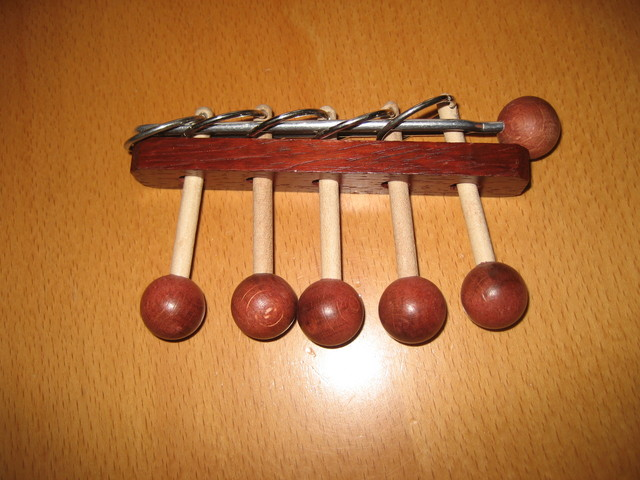In the picture, a typical wooden version of CR with 5 rings is shown. Each ring may be positioned (diagonally) on the horizontal loop or off the loop. These states may be denoted as: 1 - on, 0 - off the loop, so in this example we have:

• m=5, the puzzle contains 5 rings and
• n=2, the puzzle is binary
and the uniform condition for moving a ring is: The k-th ring can be moved between 1 and 0, if and only if ring (k—1) is in position 1 and all rings to the left of (k—1) are in position 0.

To conclude the matching of the particular classic CR puzzle of this example with our definition of a CR recursive puzzle, we note that there exist many different classic CR versions, with various number of rings, that correspond to the different values of m in our definition of CR recursive puzzles.

#### 3.2.2 SpinOutIn this puzzle, we have a slider carrying a line of discs. Each disc can be either in a vertical position (denoted by 0) or horizontal position (denoted by 1). This puzzle is equivalent to CR, if we restrict the moves to the ones that are used in the solution: From the vertical position, each disc can be turned left into the horizontal position, or to the right to a different horizontal position. We disregard this (right turned) position, as it is not needed for solving the puzzle. To see the uniform condition for this puzzle, we have a look at the pictures above and note: A disc can be turned between 0 and 1, if the disc immediately to the right is 0 (in vertical position) and all discs further right are 1 (in horizontal position). For the solution, two additional restrictions are implemented: Only a disc at the position with the additional space (second from the right) can be turned. Discs can only be moved out to the right when in position 1 (horizontal).

#### 3.2.3 Crazy Elephants Dance

The Crazy Elephant Dance is a generalized version of SpinOut. Instead of discs, we have a line of 5 elephants on a slider, and each elephant has three possible states: 0 - facing upwards, 1 - facing to the right, and 2 - facing downwards.The uniform condition is split into two parts in this case: 1. An elephant (the second from the right in the picture) may move between 0 and 1, if the one immediately to the right is in position 2, and (not shown in the picture) all further right are in position 2.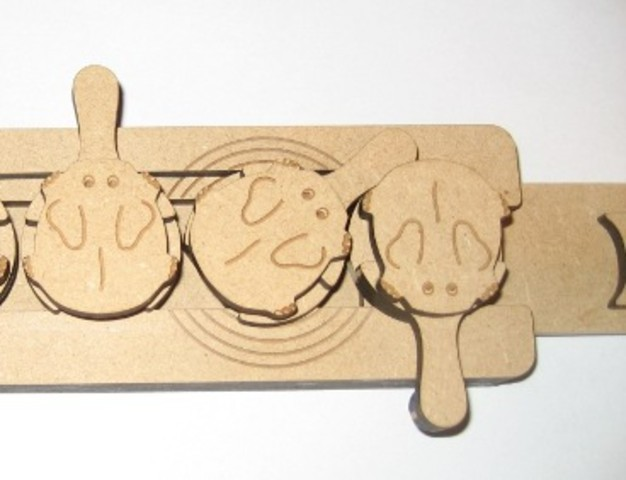2. An elephant (again the second one) may move between 1 and 2, if the one immediately to the right is in position 0, and (not shown) all further right are in position 2.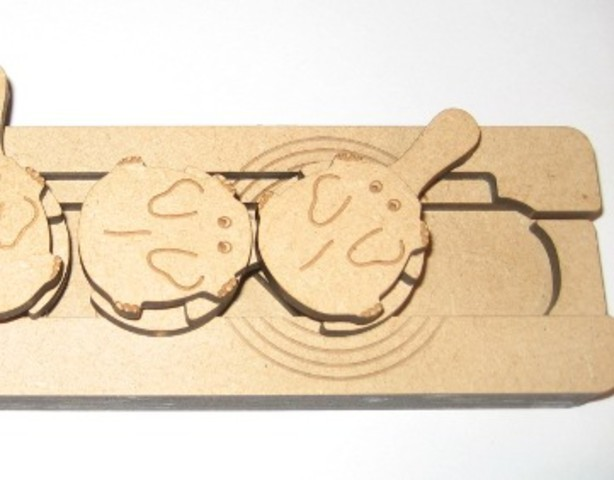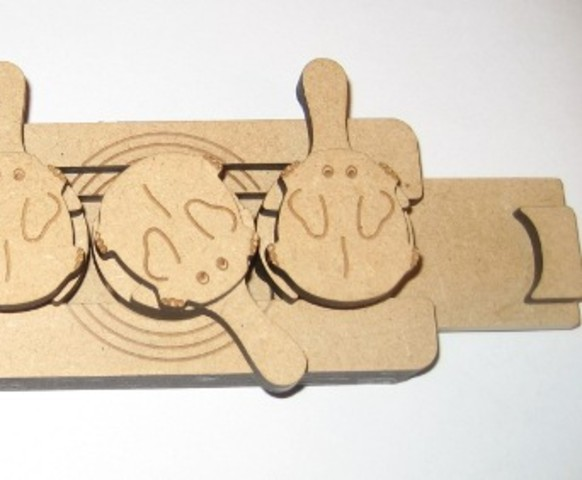Again, as for the SpinOut, the second part of the conditions above arises from the fact that the slider and elephants may only move out to the right when in position 2.

The pictures demonstrating the two parts of the condition also show an example how to move the second elephant from 0 to 2: It first has to be moved from 0 to 1 (first two pictures), then the elephant to the right of it is moved from 2 to 0 (third picture), then the second elephant may finally move from 1 to 2. This gives an idea what is necessary to move the leftmost elephant from 0 to 2, which is a vital step in the solution sequence.

#### 3.2.4 Kugellager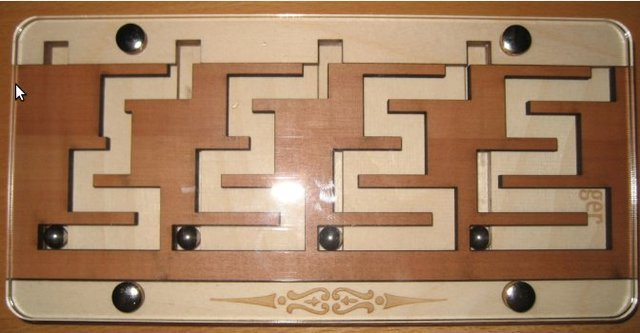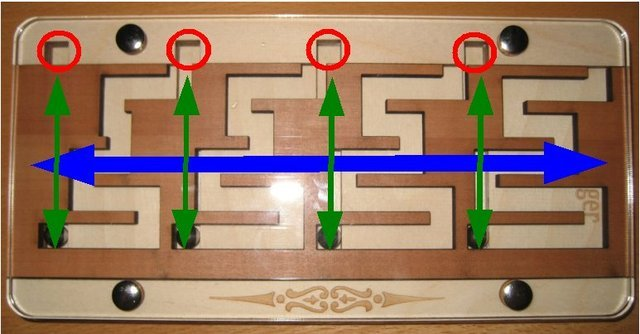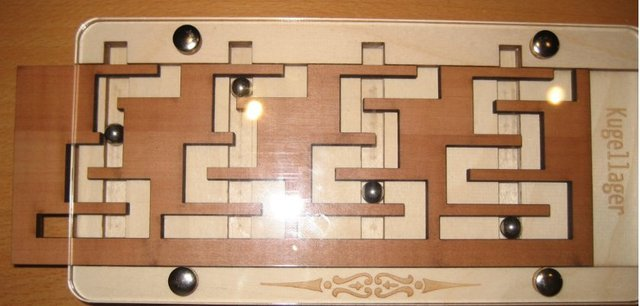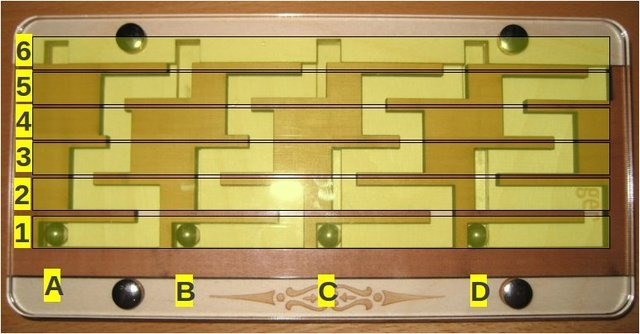The Kugellager has four balls, which are the special pieces in our definition and one slider containing some mazes for these balls. The four balls move up and down (maze in the slider permitting, green arrows), and the slider moves left and right (blue arrow). Each of the balls has 5 regular positions {0,1,2,3,4} (or {1,2,3,4,5} in the picture), and then there is an additional position 5 (or 6 in the picture), which can only be used if all balls are already in position 4, to remove the slider afterwards. This is why we may disregard this position 5.

The pictures are taken from the article  which also describes the movement in more detail and also lists the uniform condition for ball movement. Shortly summarized, a ball may move between positions i and (i+1), if all balls to the left are in a certain set of positions and all balls to the right are in a certain (different) set of positions.

This puzzle can not only be generalized to more balls, as the definition requires, but also to a higher or lower level, as the Kugellager 7 puzzle (n=7), or the Auf dem Holzweg puzzle (n=3) show.

#### 3.2.5 Tower of Hanoi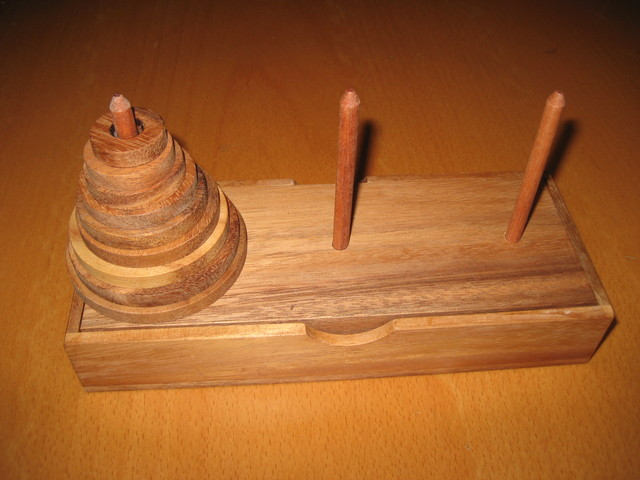At first sight, Tower of Hanoi looks very different from the puzzles we have seen so far, but it is a CR recursive puzzle and complies to our definition: It has m discs (m=9 in the picture), and each of the discs can be on one of the three piles built on the poles, so n=3. There are different variants with different numbers of discs, so the generalization to other values of m (even other values of n with more poles) is easy. All these variants will have to obey simple rules: Move only one disc at a time, and only the top disc of a tower, and a disc may only be laid down on discs that are bigger than itself. The rules deliver the uniform condition we need for our CR recursive definition and can also be translated to: To move a disc, all smaller discs have to be on a pole different from the start and destination positions of the move.

### 3.3 What does "uniform" mean in the definition?

The uniform definition covers a property that is independent of the actual number of pieces and also allows the same condition to apply to each one of them. This condition states that for all suitable indices i and j, piece number i can move between positions j and j+1 if and only if the "lower" pieces (left of i) and "higher" pieces (right of i) are in certain positions. This works independently of i: No matter if the second piece (i=2) or the fifth piece (i=5) is to be moved.

There are also puzzles for which the "higher" pieces are irrelevant, for example:

• SpinOut: In the example above we have seen that to toggle a piece (vertical ↔ horizontal), the piece one below must be vertical and all below that must be horizontal. This is the uniform condition for the lower pieces, the higher ones do not matter.
• Kugellager: In article  the conditions for ball movement are sketched and depend on the source and destination positions of the current move. We just take out one example of the conditions in this article. It states, if a ball moves between positions 1 and 2, all lower balls have to be in position 5, and all higher balls have to be in position 1, 2, or 5. This rule holds for all balls, so it is a uniform condition.

### 3.4 Where does the term "recursive" come from?

All puzzles contained in this compendium allow a recursive description of their solution. As an example, take the Tower of Hanoi. This puzzle has three positions, two of which are initially empty and one carries a stack of discs that are ordered from biggest in the bottom to smallest on top. The aim of the game is to move the stack of discs to the third position (whichever that may be) obeying the following two rules:

1. Only one disc may be moved at a time
2. Never place a bigger disc on top of a smaller oneA typical Algorithm to solve this problem can be described informally as follows:

 Move Tower of n discs from startTower to endTower: 6 - startTower - endTower → auxiliaryTower // the tower that is not one of the two above if n>1 then Move Tower of n—1 discs from startTower to auxiliaryTower Move one disc from startTower to endTower if n>1 then Move Tower of n—1 discs from auxiliaryTower to endTower

This algorithm works by moving the n—1 top discs away on the auxiliary tower, then disc n, then the ones on the auxiliary tower to the destination tower.

What algorithm do we use in lines 3 and 6 in order to move an n—1 disc tower? It is our very same algorithm, that calls itself recursively, and now we have our justification to call this puzzle "recursive", as it can be solved by such a recursive algorithm. More details about Tower of Hanoi can be found e.g. here: 

While for this puzzle it may seem obvious, the question remains for the other puzzles: Why are the other puzzles in this compendium also recursive?

Well, this drills down to the core of the matter. What do all these puzzles have in common, and how are they related to the "classic" Chinese Rings puzzle? A first insight might be to look at a solution method for Chinese Rings. The goal of this puzzle is to remove all the rings from the loop.This can be established by a recursive algorithm with the following ideas:

 move i-th ring off the loop: Move ring i—1 (to the left of ring i) onto the loop move all rings left of i—1 off the loop then perform the movement of i-th ring move i-th ring onto the loop: Move ring i—1 (to the left of ring i) onto the loop move all rings left of i—1 off the loop then perform the movement of i-th ring
Here we see that for the movement of the i-th ring, preparation moves must be performed, and for these the algorithm can invoke itself recursively. More details can be found in the book  and a more mathematical observations in the book .

### 3.5 Tuple Representation and a Different Notation: n-ary Puzzles

Before the formalization of the recursive puzzles as above, a different notation was used in prior discussions: "n-ary Puzzles". This notation has its roots in well known puzzles: Chinese Rings, The Brain, SpinOut, The Key, and Binary Burr. These are typically called "binary" puzzles, because their "ring pieces" have two different states each, and their solution length function are asymptotically 2m. Ternary Burr, Tern Key, Crazy Elephant Dance are called "ternary" puzzles because they generalize the binary concepts to pieces having three different states. However, interestingly enough, their solution length functions are still asymptotically 2m, which is a justification for our structural definition. Why ternary puzzles can have 2m as solution length function is discussed below.

A mathematical argument for calling these puzzles "n-ary" is that their current state can be represented by an m-tuple of entries ranging in {0,...,n—1}. For example, the SpinOut starting configuration would be: (0,0,0,0,0,0,0) and the goal configuration: (1,1,1,1,1,1,1). A solution could then be described as a sequence of such tuples:

(0,0,0,0,0,0,0) → (0,0,0,0,0,0,1) → (0,0,0,0,0,0,1,1) → ... → (1,1,1,1,1,1,0) → (1,1,1,1,1,1,1)

A ternary example is the Crazy Elephant Dance, with the following encoding:

(0,0,0,0,0) → (0,0,0,0,2) → (0,0,0,1,2) → (0,0,0,1,0) → (0,0,0,2,0) → (0,0,0,2,2) → (0,0,1,2,2) → ... → (2,2,2,2,2)

### 3.6 Puzzle Parameter and Different View: Number of Moves

You may have noticed that In the definition of CR recursive puzzle we did not refer to the number of moves required to solve the puzzles, which is a central property in a different definition of the class of puzzles in this compendium (see discussion below). The number of moves seems related to the parameters in our definition, but no uniform relation has been determined for all the puzzles of this compendium, and this seems impossible, as we will see: The key count is the number of moves that the special pieces (or "ring pieces") move during the solution process. Similar to approaches in Computer Science, we will sometimes not provide an exact function of the number of moves, but will use an approximate notation. In such cases the exact number of moves might not have been calculated yet, but by analogy one has a strong indication what it could be like.

This notation describes the asymptotic growth and we are only interested in the fastest growing elements of this number of moves function. Following are some examples for this notation:

Exact function Approximation Note
2m+m+3 Θ( 2m ) Linear and constant summands neglectable
2 · 5n Θ( 5n ) Constant factor neglectable
2(m—1) Θ( 2m ) 2(m—1)= (1/2)·2m, constant factor neglectable
3 · (2m—1) — 2·m Θ( 2m ) Combination of examples above

The Θ notation is taken from Computer Science and Mathematics, the formal definition and details are explained in .

### 3.7 CR recursive puzzles with solution length only ~2m

Why do some CR recursive puzzles with n>2 have a solution with only ~2m moves, not ~nm? CR recursive puzzles with solution length ~nm obviously use all different combinations of piece positions in their solution. To see this, just recall that the number of m-tuples over a set with n different values is exactly nm and so all these tuples occur in the description of the solution (see tuple representation above). So the puzzles in question that have solution length ~2m will not use all these tuples in their solution, but leave some out. For example, on the (shortest) solution path for the Ternary Burr, you will never find a configuration corresponding to tuple (1,0,0,0) -- this configuration is not needed. If you have this puzzle or the equivalent Crazy Elephant Dance, just try it out (with the lowest 4 elephants)! When you try the solution for on of these puzzles, you will find that directly before reaching this position, you will have the configuration (1,0,0,1) -- which is not part of the shortest solution either -- and this will be the only successor configuration after (1,0,0,1). Shortly said: only from (1,0,0,1) you reach (1,0,0,0), and your only option is going back to (1,0,0,0), and this is a detour way back from the solution from (0,0,0,0) to (2,0,0,0). Please see picture for these solution steps in the actual puzzle.This effect occurs in several different puzzles, but what are the reasons for some puzzles to use all possible configurations, and for some others to omit configurations in their solution? Several reasons have been observed so far:

1. Condition for moves: Puzzles with all configurations (and hence solution length nm) have conditions that involve both lower and higher pieces. For an example see the Kugellager above. Others do only involve a part of the other pieces. The examples in this paragraph are of such nature. For Ternary Burr and Crazy Elephant Dance, it only matters which positions the lower pieces have. Pieces may be moved no matter in which positions the higher pieces currently are. This allows to bring a piece from position 0 to n—1 without touching the higher pieces at all. When solving Kugellager, you will notice that when moving the lowest piece later in the solution, you will have to move higher pieces before.
2. The second observation deals with Tower of Hanoi and Rudenko Clips. Both have the same general structure (3 positions) and equivalent rule:
• Tower of Hanoi: bigger disc may not be on smaller one
• Rudenko Clips: smaller clip may not be around bigger one
However, the first one has a solution length ~2m, while the Clips need ~3m moves. Here, the condition is the same, but there is an additional condition in Rudenko Clips: The three positions 0, 1, and 2 are in a row and a clip may only move between positions 0 and 1, if the stack of smaller clips is on 2, and a clip may only move between positions 1 and 2, if the stack of smaller clips is on 0. No direct move from 0 to 2 is possible for all clips except the biggest one. For Towers of Hanoi, we may use positions freely (only obeying the "bigger disc" rule). In the picture below, the red clip is not able to move from position 0 to 2 over the green one, while this would be the canonical next step in Tower of Hanoi. For a discussion on graph representations also leading to 2m and 3m as solution lengths, please see .### 3.8 Tower of Hanoi - binary or ternary?

In the paragraphs above the relation between binary and ternary regarding the solution length has been discussed. There is also a binary representation that can be used for describing the solution of Tower of Hanoi, for details, please see .

Recently Goh Pit Khiam created a nice illustrated example of a variant of Tower of Hanoi: Linear Tower of Hanoi. In this variant, the three poles are in a line and a disc may only move from a pole to an adjacent pole. The obvious implications are that there are no direct moves between pole 0 and 2, and that a bigger disc may not move between poles 0 and 2, whenever there is a smaller one on pole 1. This makes it very similar to a Rudenko Clips (see previous section above). The less obvious implication is that the puzzle follows a ternary gray code. Please see  for the illustrated example of this puzzle, which also shows a nice ternary representation of Tower of Hanoi.

### 3.9 A different definition for n-ary puzzles based on number of moves

Our definition of CR recursive puzzle is based on the structure of the puzzle, which makes it (relatively) easy to spot if a puzzle belongs to this class. However, there is another different and commonly used definition of n-ary puzzles that first requires the puzzle to be analyzed and solved fully before it can be classified. Once one has determined that there are m special pieces and the solution length function is asymptotically equal to nm, it is classified as n-ary (in this solution length based notation). We are using the structural definition provided above (as it seems easier to apply it in most cases), but there might be some confusion. Some ternary puzzles (our definition) may have a solution with (asymptotic) length 2m, while the solution length function based definition would call them "binary" for this reason. One prominent example is the Ternary Burr by Pit Khiam Goh. This ternary (sic!) variation of the Binary Burr by Bill Cutler would be classified as binary following the solution length based definition.